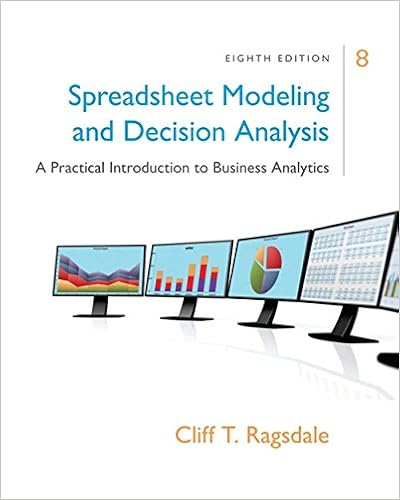# 3 the cdf of a continuous random variable can show

• Test Prep
• 7
• 100% (1) 1 out of 1 people found this document helpful

This preview shows page 2 - 4 out of 7 pages.

##### We have textbook solutions for you!
The document you are viewing contains questions related to this textbook.The document you are viewing contains questions related to this textbook.
Chapter 2 / Exercise 7
RagsdaleExpert Verified
______ 3. The CDF of a continuous random variable can show increases and decreases. _____ FALSE______ 4. The median is equal to the expected value for all continuous random variables. Write the letter of your choice on the line before the question number.
5. Let ?~𝑁(? = 3, 𝜎 = 2), ?~𝑁(? = 7.5, 𝜎 = 3)be independent normal random variables. Another random variable Y is defined as ? = −? + ?. What can be determined about the distribution of Y? (3 points)
A random variable, X, has a normal distribution with a mean of 132 and a variance of 49. Answer questions 6-7 below using this information and the Empirical Rules. __B__6. What is the probability that X is between 132 and 146? (2 points)
7.What is value of the 84thpercentile of this distribution? (2 points)
##### We have textbook solutions for you!
The document you are viewing contains questions related to this textbook.The document you are viewing contains questions related to this textbook.
Chapter 2 / Exercise 7Expert Verified
•••